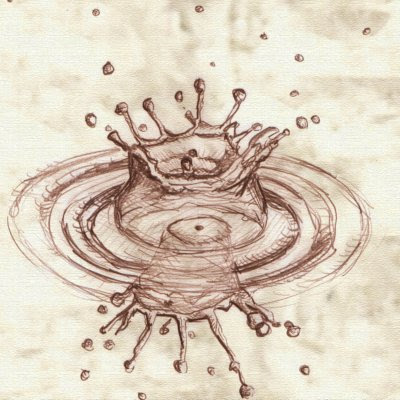### The boson, the spin and the gravitonSome days ago, ATLAS has been released a draft about the spin of the new boson. The decay channels studied are the fab four: $H \rightarrow \gamma \gamma$, $H \rightarrow WW^*$, $H \rightarrow l\nu l\nu$, $H \rightarrow ZZ^* \rightarrow 4l$. The idea is combining data from the four channels in order to understand the spin of the new boson, in detail to distinguish between two cases: spin 0 ($J^P = 0^+$), and so a boson compatible with the Standard Model, and spin 2 ($J^P = 2^+$), that it could be connected with a model (arXiv) that represents a light coupling between the Standard Model's fields and the hypothetical graviton.
These the ATLAS' conclusions:
The data are in good agreement with the expected distributions of a $J^P=0^+$ particle while the graviton-inspired $J^P=2^+$ model, that is expected to be produced dominantly via the gluon fusion process, is excluded at more than 99.9% conﬁdence level.
We could say that it starting the elimination process of the models that would lead the research of the new physics beyond the Standard Model for the next years. A good luck to all of them, but we don't forget the key role of the Standard Model, that is in some sense confirmed by this last draft from ATLAS.